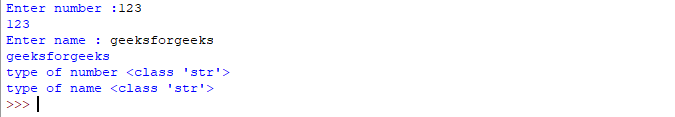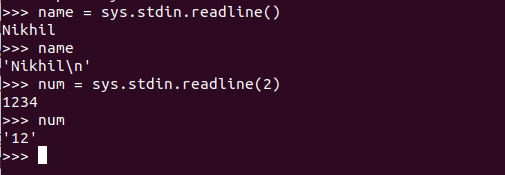# Difference between input() and sys.stdin.readline()

• Difficulty Level : Easy
• Last Updated : 29 Oct, 2021

Python is a widely used general-purpose language that can be used for many purposes. Taking input in any language is as important as breathing for humans. Python provides various methods for taking input. However, we all may get confused about how each method is different from one another. In this article, we will discuss about two such methods i.e `input()` and `sys.stdin.readline()`.

Note: For more information, refer to Python Tutorial

## Input()

This function first takes the input from the user and then evaluates the expression, which means Python automatically identifies whether the user entered a string or a number or list. If the input provided is not correct then either syntax error or exception is raised by Python.

How the input function works in Python :

• When `input()` function executes program flow will be stopped until the user has given input.
• The text or message display on the output screen to ask a user to enter input value is optional i.e. the prompt, will be printed on the screen is optional.
• Whatever you enter as input, input function converts it into a string. if you enter an integer value still input() function converts it into a string. You need to explicitly convert it into an integer in your code using typecasting.

Example:

 `# Program to check input  ``# type in Python ``   ` `num ``=` `input` `(``"Enter number :"``) ``print``(num) ``name1 ``=` `input``(``"Enter name : "``) ``print``(name1) ``   ` `# Printing type of input value ``print` `(``"type of number"``, ``type``(num)) ``print` `(``"type of name"``, ``type``(name1))`

Output:Stdin stands for standard input which is a stream from which the program reads its input data. This method is slightly different from the input() method as it also reads the escape character entered by the user. More this method also provides the parameter for the size i.e. how many characters it can read at a time.

Example:

 `# Python program to demonstrate``# sys.stdin.readline()`` ` ` ` `import` `sys`` ` `name ``=` `sys.stdin.readline()``print``(name)`` ` `num ``=` `sys.stdin.readline(``2``)``print``(num)`

Output: